# 概述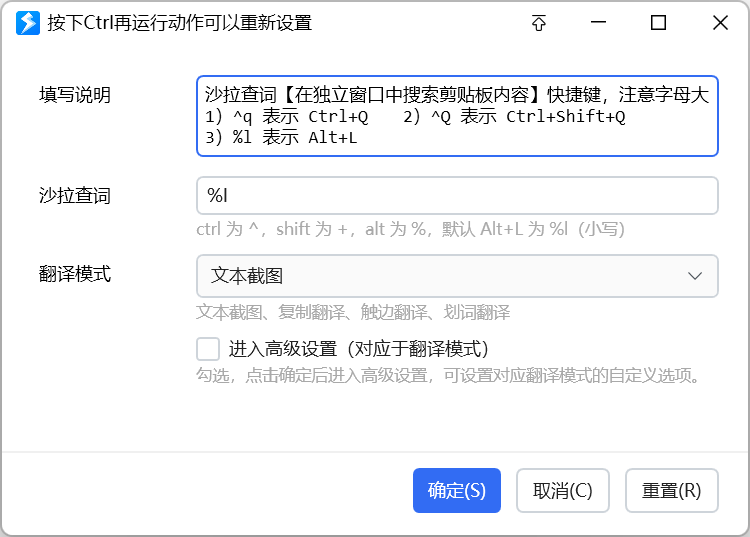## 工作模式

【编辑动作变量的值】

【编辑词典数据】自1.34.5版本增加。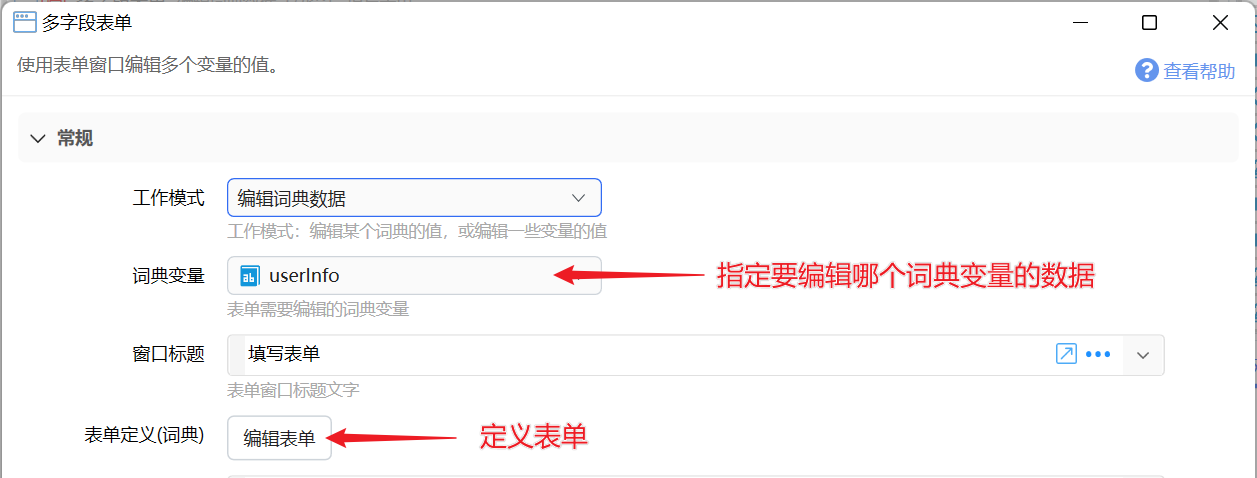【编辑词典数据（动态）】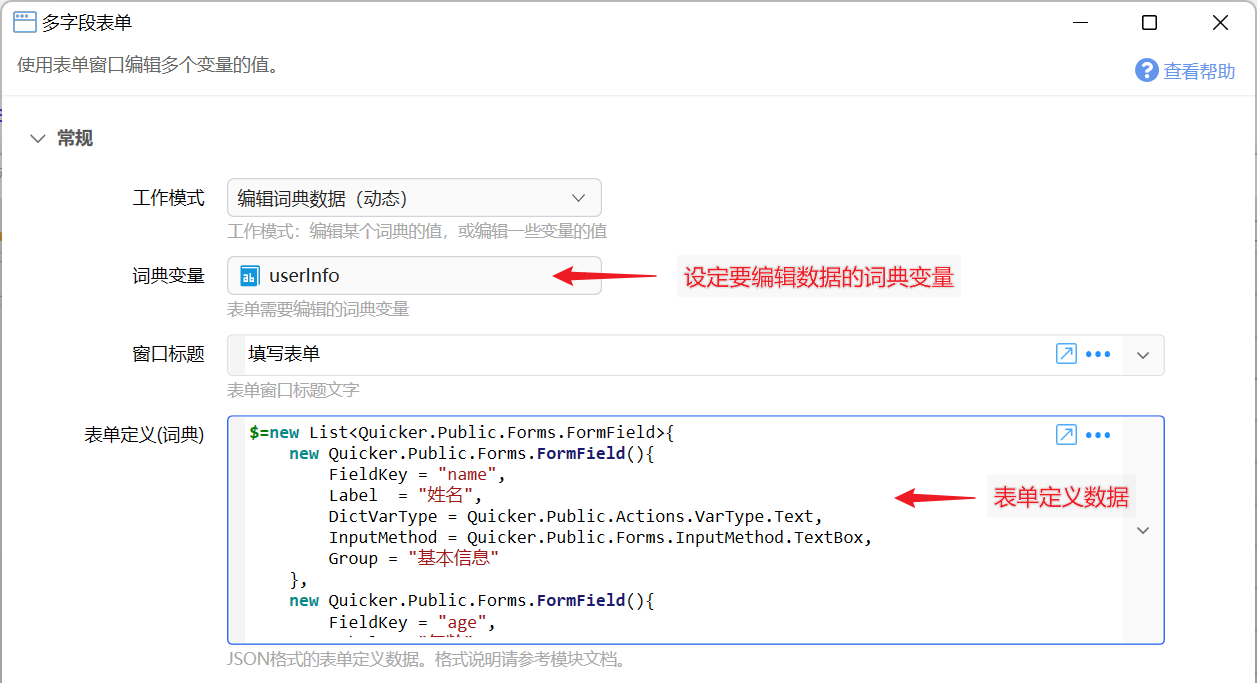## 使用

• Tab：跳转到下个控件
• Shift+Tab：跳转到上个控件
• Ctrl+Tab 或 Ctrl+↓：跳转到下一个字段
• Ctrl+↑：跳转到上一个字段
• Alt+S：保存
• Alt+C、Esc：取消
• Alt+R：重置表单内容

# 参数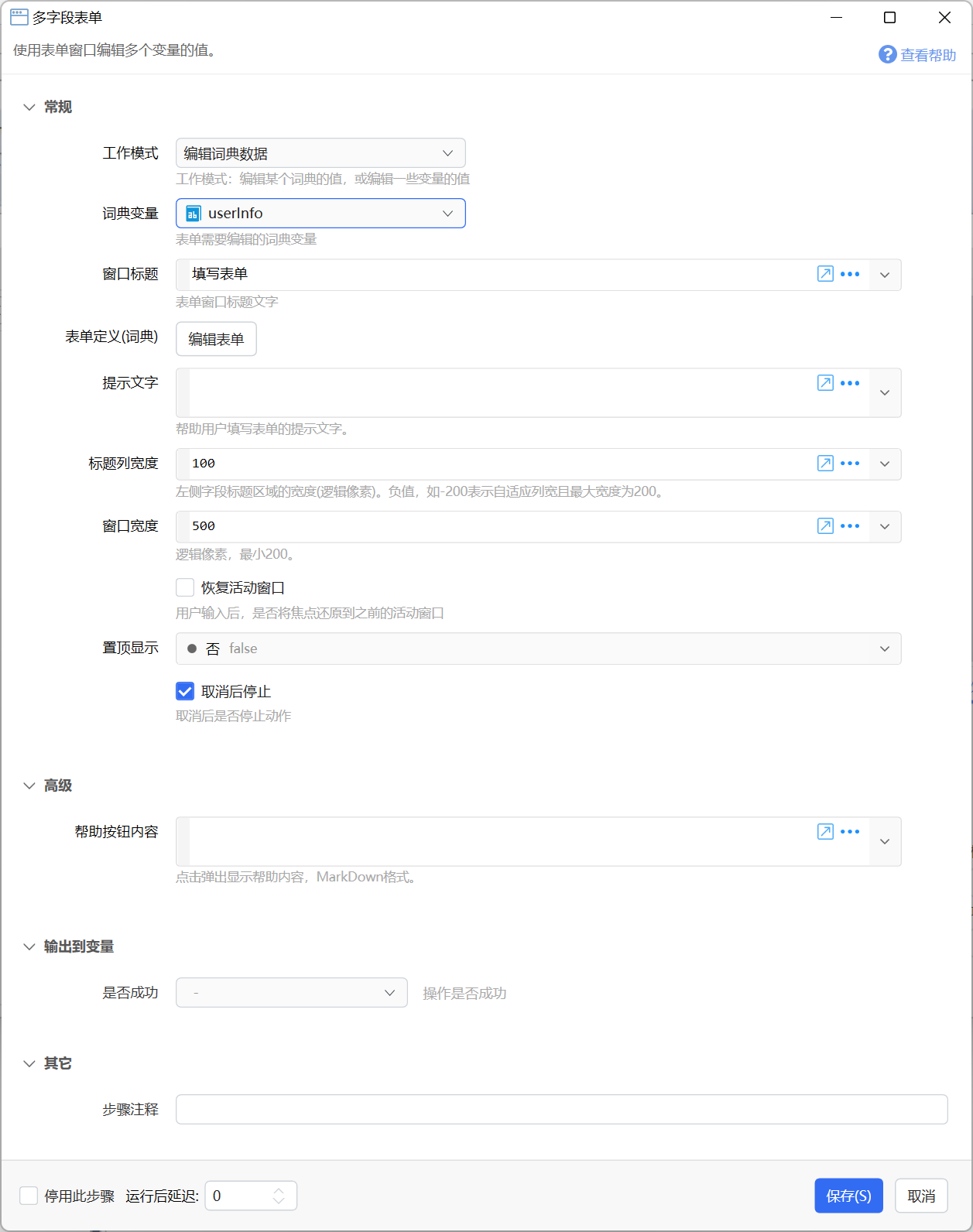【工作模式】选择用途：编辑变量，还是编辑某个词典的数据。

【词典变量】工作模式为“编辑词典数据”时，指定需要被编辑数据的词典类型变量。

【窗口标题】表单窗口的标题文字。

【表单定义】打开表单定义窗口设计表单。动态表单的数据定义格式请参考本文下面部分的章节。

【提示文字】显示在表单下方的提示文字。

【标题列宽度】设置表单文本标题部分所占宽度。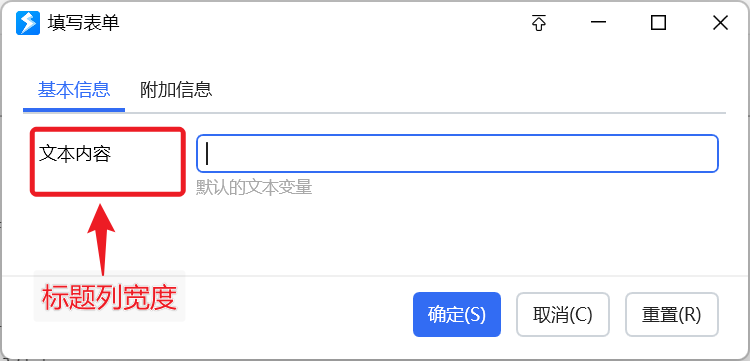【窗口宽度】表单窗口的整体宽度。

【置顶显示】是否自动置顶表单窗口。

【恢复活动窗口】在表单关闭后，是否将输入焦点恢复到显示表单之前的窗口上。

【取消后停止】取消表单后，是否停止动作的后续步骤。

# 设计表单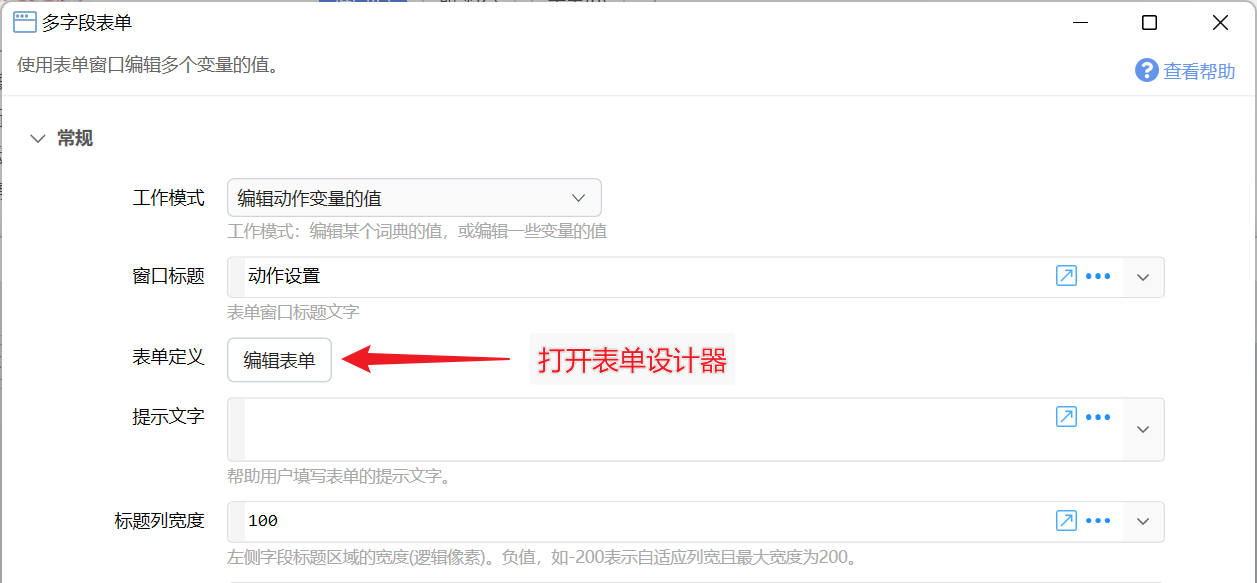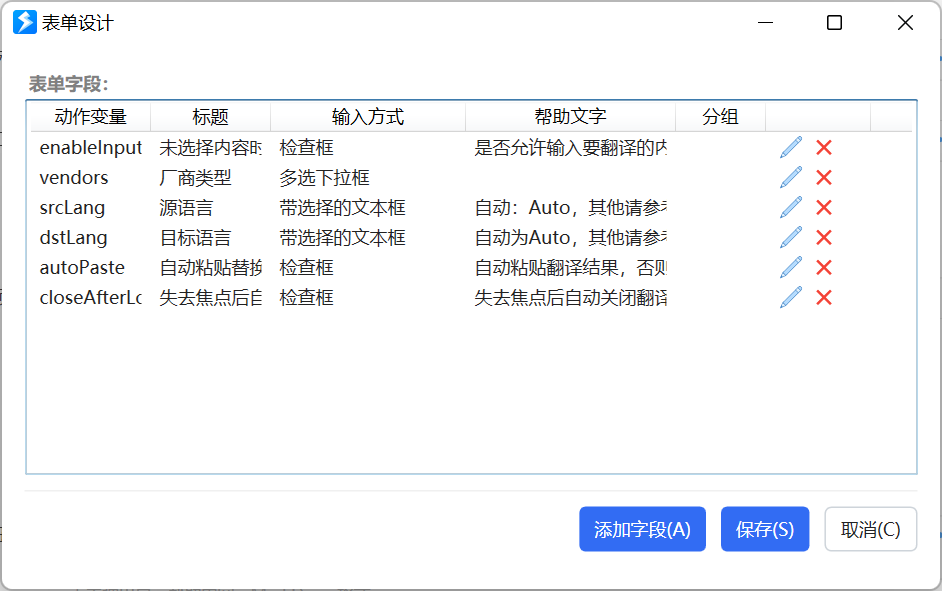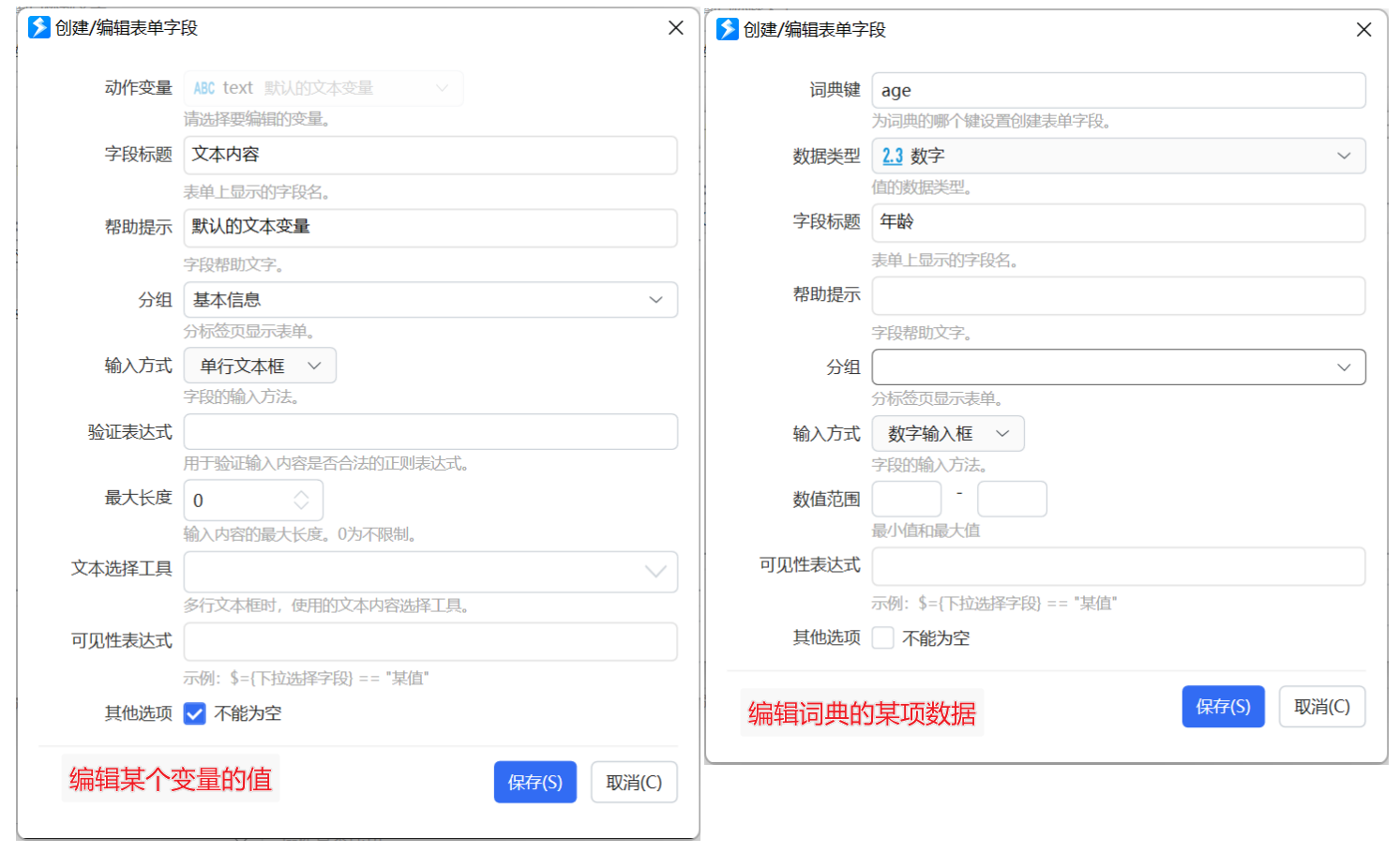【动作变量】工作模式为“编辑动作变量的值”时，设定要编辑的变量名。

【词典的键名】工作模式为“编辑词典数据”时，指定要编辑词典的哪个(key)对应的值。

【数据类型】工作模式为“编辑词典数据”时，指定词典键所对应的值的数据类型。例子：加入某个存储用户信息的词典，有“姓名”和“年龄”两个键，则它们对应的数据类型分别为 文本 和 数字。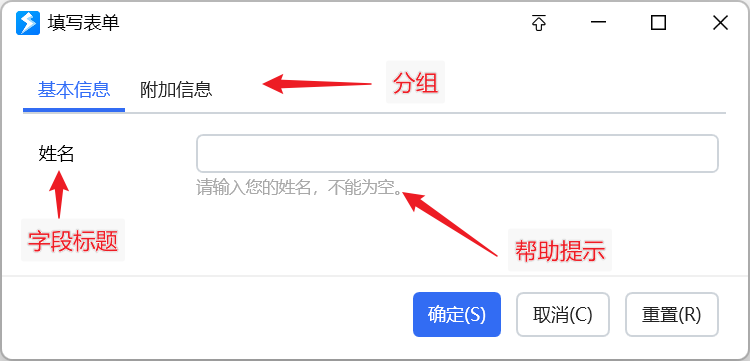【字段标题】显示在表单左侧的字段名称。

【帮助提示】显示在输入框下方的提示文字。

【分组】字段较多时，可以按逻辑关联将某些字段放置在分组中。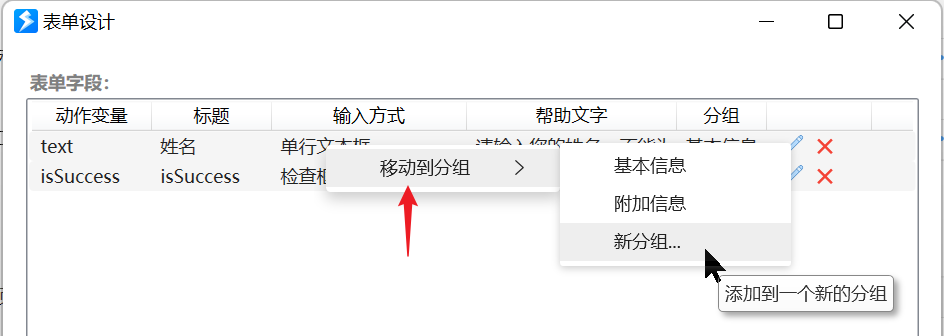【输入方式】设定使用哪种控件编辑值。

【验证表达式】输入方式为文本框时，对输入内容进行校验的正则表达式。

【最大长度】允许输入的最多字符数。

【数值范围】输入内容的最小值和最大值（可选）。

【文本选择工具】在输入框右侧显示的快捷选择按钮。

【可见性表达式】依据某些‘检查框’和‘下拉框’方式输入的字段值，更新当前字段的可见性。

• 操作类型为“编辑动作变量的值”时，可见性表达式中可以使用当前主程序或子程序的其它变量。如`\$= {铃声模式变量} == "自定义"`中，`铃声模式变量`为另外一个变量。
• 操作类型为“编辑词典数据”时，可见性表达式中可以将其它词典键当做变量访问。如` \$= {铃声模式}.ToString()=="自定义" `中，`铃声模式`为当前被编辑词典的另外一个键。

【控件宽度】可选。输入框宽度，为逻辑像素值（实际像素需要再乘以屏幕缩放比例）。

【不能为空】是否必须输入内容。

### 分割线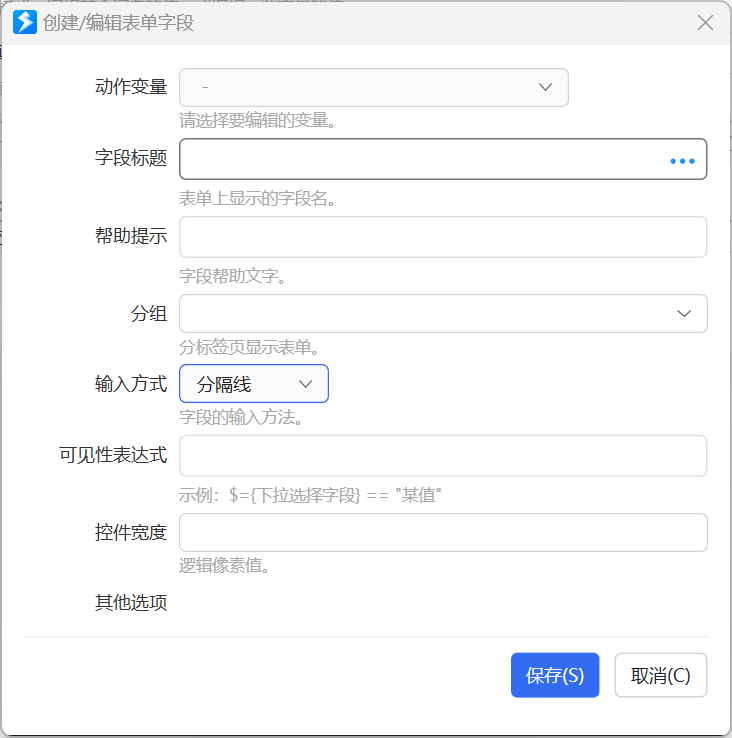(1.38.38+版本)将分割线的字段标题设置为`[]`，可以隐藏分割线，只显示一个空白行。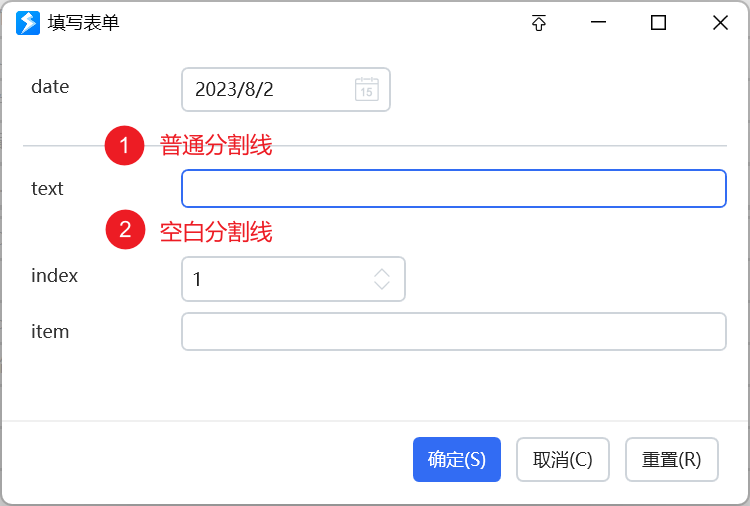# 动态表单定义数据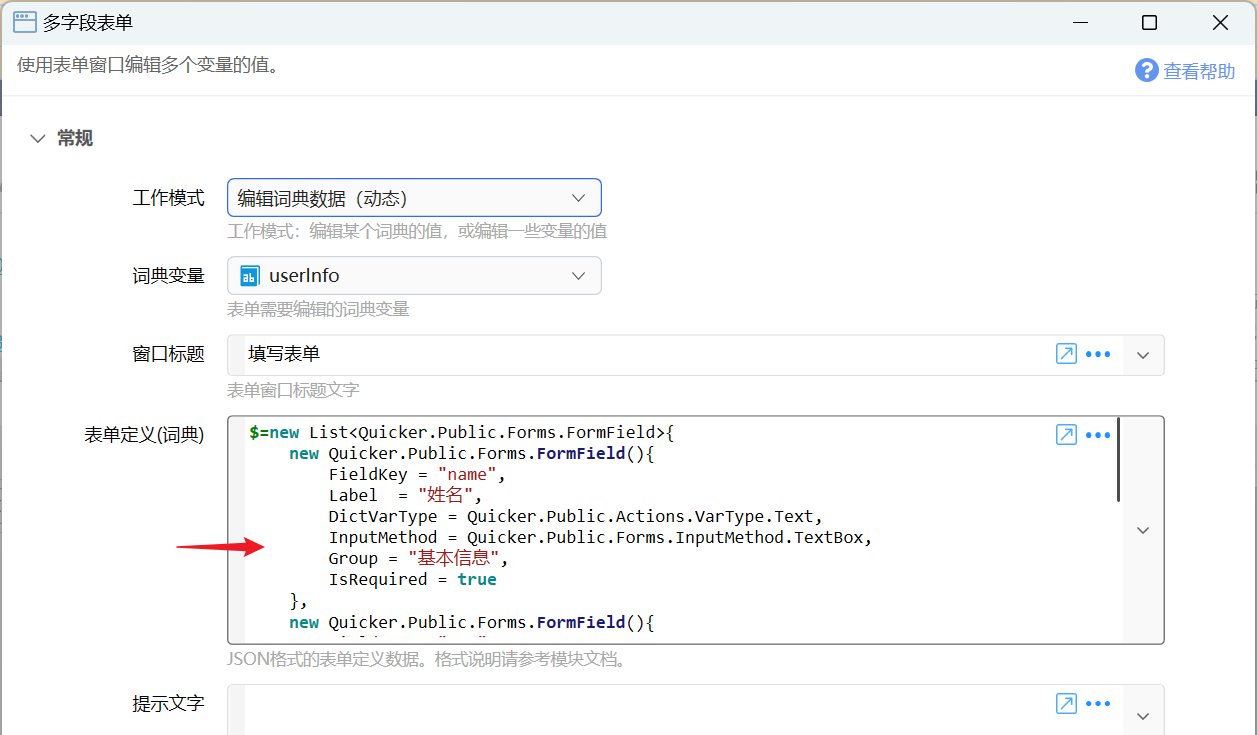FormField类型的定义如下：

``````
namespace Quicker.Public.Forms
{
/// <summary>
/// 表单字段
/// </summary>
public class FormField
{

/// <summary>
/// 对应的变量
/// </summary>
public string FieldKey { get; set; }

/// <summary>
/// 对于编辑词典键值的情况，设定类型
/// </summary>
public VarType? DictVarType { get; set; }

/// <summary>
/// 字段名、标签
/// </summary>
public string Label { get; set; }

/// <summary>
/// 帮助文本
/// </summary>
public string HelpText { get; set; }

/// <summary>
/// 帮助链接
/// </summary>
public string HelpLink { get; set; }

/// <summary>
/// 输入方法
/// </summary>
public InputMethod InputMethod { get; set; }

/// <summary>
/// 选项内容。使用换行分隔多条。
/// </summary>
public string SelectionItems { get; set; }

/// <summary>
/// 是否必填
/// </summary>
public bool IsRequired { get; set; }

/// <summary>
/// 最小值
/// </summary>
public string MinValue { get; set; }

/// <summary>
/// 最大值
/// </summary>
public string MaxValue { get; set; }

/// <summary>
/// 验证规则表达式
/// </summary>
public string Pattern { get; set; }

/// <summary>
/// 最大长度
/// </summary>
public int MaxLength { get; set; } = 0;

/// <summary>
/// 输入法状态控制
/// </summary>
public string ImeState { get; set; }

/// <summary>
/// 文本选择工具：以英文半角逗号分隔的工具名。
/// </summary>
public string TextTools { get; set; }

/// <summary>
/// 可见性表达式
/// </summary>
public string VisibleExpression { get; set; }

/// <summary>
/// 分组名称
/// </summary>
public string Group { get; set; }

}
}
``````

``````namespace Quicker.Public.Actions
{
/// <summary>
/// 参数类型
/// </summary>
public enum VarType
{
/// <summary>
/// 文本
/// </summary>
[Display(Name = "文本", Order = 10)]
Text = 0, //文本

/// <summary>
/// 数字（小数）
/// </summary>
[Display(Name = "数字(小数)", Order = 2)]
Number = 1, // 数字

/// <summary>
/// 数字（整数）
/// </summary>
[Display(Name = "数字(整数)", Order = 3)]
Integer = 12, //整数

/// <summary>
/// 布尔(是否)
/// </summary>
[Display(Name = "布尔(是否)", Order = 1)]
Boolean = 2, //布尔

/// <summary>
/// 文本列表
/// </summary>
[Display(Name = "文本列表", Order = 21)]
List = 4, //字符串列表

/// <summary>
/// 时间日期
/// </summary>
[Display(Name = "时间日期", Order = 11)]
DateTime = 6, //日期时间
}

}``````

InputMethod的定义（已去除一些在表单中不支持的类型，在Json中写对应的数字值）：

``````namespace Quicker.Public.Forms
{
/// <summary>
/// 输入方式
/// </summary>
public enum InputMethod
{
/// <summary>
/// -无-
/// </summary>
[Display(Name = "-无-")]
None = 0,

/// <summary>
/// 单行文本框
/// </summary>
[Display(Name = "单行文本框")]
TextBox=1,

/// <summary>
/// 多行文本框
/// </summary>
[Display(Name = "多行文本框")]
TextEditor = 2,

/// <summary>
/// 下拉选择框
/// </summary>
[Display(Name = "下拉选择")]
DropDown = 3,

/// <summary>
/// 滑块
/// </summary>
[Display(Name = "滑块")]
Slider = 4,

/// <summary>
/// 日期选择框
/// </summary>
[Display(Name = "日期选择")]
DatePicker = 5,

/// <summary>
/// 检查框
/// </summary>
[Display(Name = "检查框")]
CheckBox = 6,

/// <summary>
/// 数字输入框
/// </summary>
[Display(Name = "数字输入框")]
NumberBox = 7,

/// <summary>
/// 多选下拉框
/// </summary>
[Display(Name = "多选下拉框")]
CheckComboBox = 8,

/// <summary>
/// 颜色选择框
/// </summary>
[Display(Name = "颜色选择器")]
ColorPicker = 9,

/// <summary>
/// 密码框
/// </summary>
[Display(Name = "密码框")]

/// <summary>
/// 带选择的文本框
/// </summary>
[Display(Name = "带选择的文本框")]
EditableDropDown = 11,

/// <summary>
/// 字体选择器
/// </summary>
[Display(Name = "字体选择器")]
FontFamilySelector = 12,

/// <summary>
/// 带选择的文本框(支持选项筛选)
/// </summary>
[Display(Name = "带选择的文本框(支持选项筛选)")]
EditableAutoCompleteDropDown = 13,

[Display(Name = "分隔线")]
Separator = 100
}
}``````

``````namespace Quicker.Modules.TextTools
{
/// <summary>
/// 输入框工具类型
/// </summary>
public enum TextToolType
{
Na, //不支持的选项

[Display(Name = "在代码窗口中编辑")]
EditInCodeWindow, //在代码窗口中编辑

[Display(Name = "选择一个文件")]
SelectSingleFile, //选择一个文件的路径

[Display(Name = "选择多个文件")]
SelectMultiFile, //选择多个文件路径

[Display(Name = "选择文件夹")]
SelectSingleFolder, //选择一个文件夹
//SelectMultipleFolder, //选择多个文件夹

[Display(Name = "选择窗口并获取进程的路径")]
SelectProcessPath, //选择进程的完整路径

[Display(Name = "选择窗口并获取进程名称")]
SelectProcessName, //选择进程名称

[Display(Name = "选择窗口并获取标题")]
SelectWindowTitle, //选择窗口名称

[Display(Name = "选择窗口并获取其类名")]
SelectWindowClass, //选择窗口类

[Display(Name = "选择屏幕位置")]
SelectLocationPoint, //选择位置

[Display(Name = "选择屏幕区域")]
SelectLocationArea,     //选择区域

[Display(Name = "选择屏幕颜色")]
SelectColor,        //选择屏幕颜色

[Display(Name = "选择颜色(#RRGGBB)")]
ColorPicker,        //颜色选择器

[Display(Name = "选择颜色(#AARRGGBB)")]
ColorPickerArgb,        //颜色选择器

[Display(Name = "截图")]
CaptureToFile, //截图并保存到图片

[Display(Name = "选择图标")]
SelectIcon,         //选择图标

[Display(Name = "输入并获取键名")]
SelectKeyName,      // 按键名称（System.Windows.Forms.Keys)

[Display(Name = "输入并获取'模拟按键B'的值")]
SelectSendKeysData, // 选择模拟按键B的键值

[Display(Name = "输入并获取虚拟键码数字")]
SelectKeyCode, // 选择模拟按键B的键值

[Display(Name = "选择动作ID")]
SelectActionId,

[Display(Name = "选择动作名称")]
SelectActionName,

[Display(Name = "选择控件XPath")]
SelectControlXPath,

[Display(Name = "布尔表达式助手")]
BoolExpressionHelper,
//[Display(Name= "Wpf窗体设计器")]
//WindowDesigner,

[Display(Name = "选择保存路径")]
SelectSavePath, //选择一个文件夹

[Display(Name = "选择窗口句柄")]
SelectWindowHandle, //选择窗口句柄
}
}
``````

## 注意事项

`\$=_context.GetRootContext().GetVarValue("变量名")`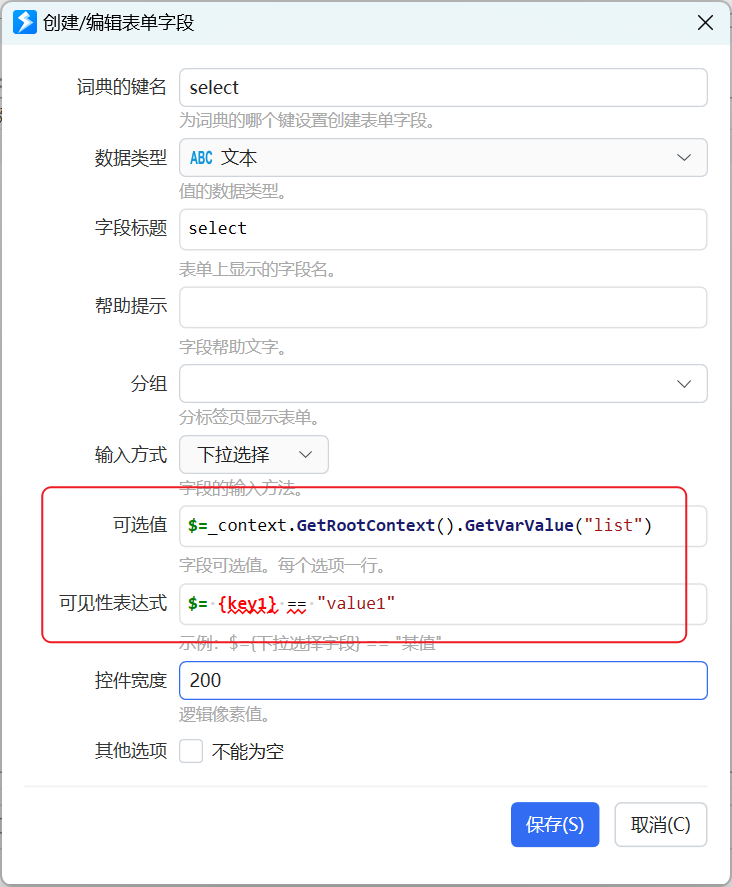# 更新历史

• 1.5.7 增加提示文字参数。
• 1.34.5 增加分组、编辑词典内容等功能。
• 20230326 增加控件宽度说明、编辑词典时使用动作变量的说明。
• 20230802 增加分割线说明。

### 反馈与讨论

KBC 9天21小时前 9天21小时前